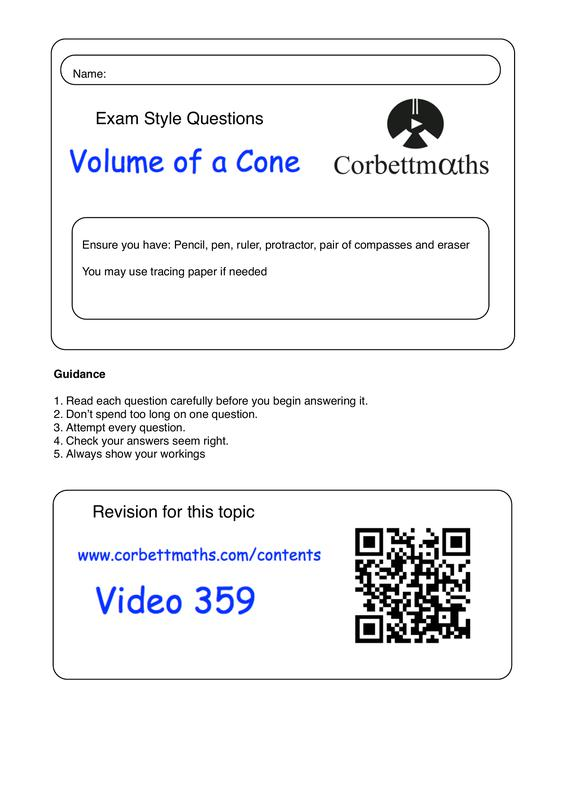GCSE Mathematics
Math Expression: Free Math Tutor Online
Learn math with free math tutor online. You'll get math videos, practical study tips and practice questions with step-by-step solutions.
Full teach, learn, methods and practice visual powerpoint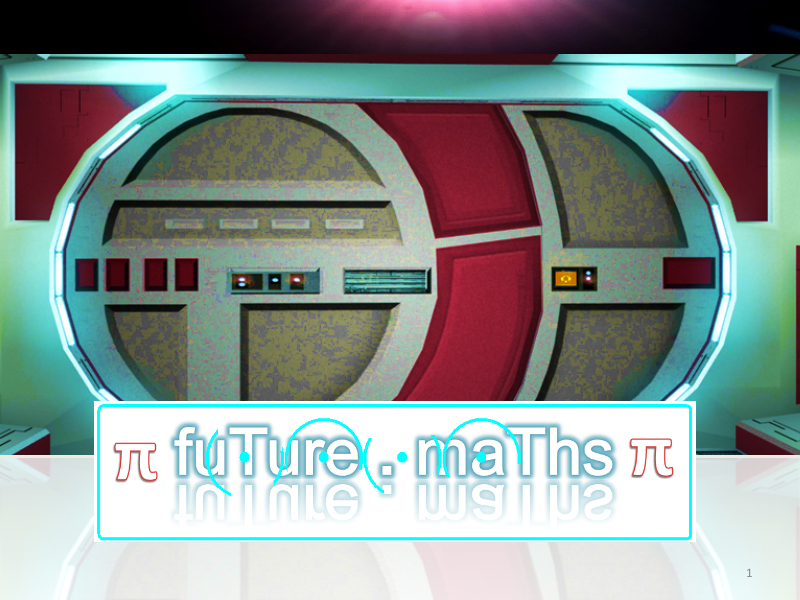Session Handout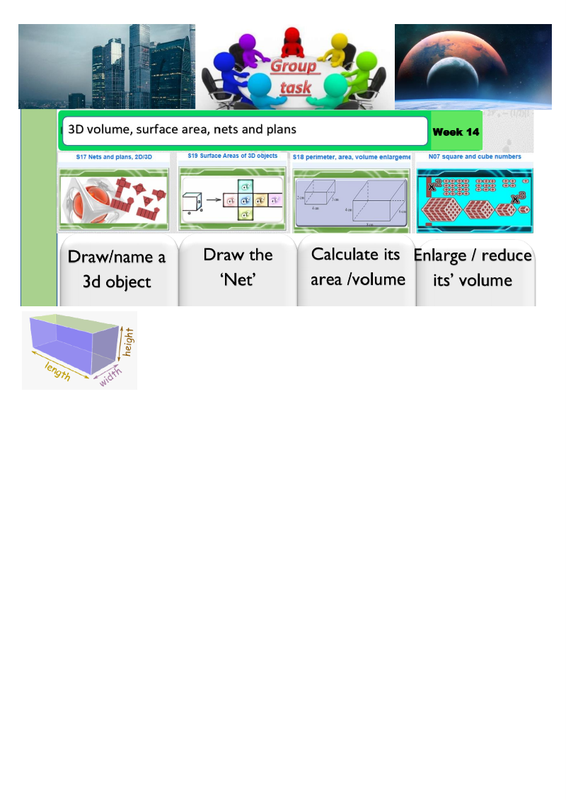Area and Surface Area ppt 2021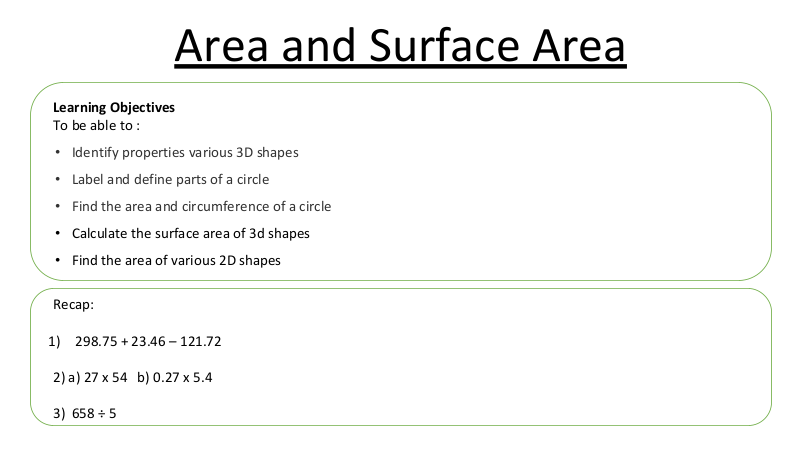2d shapes - Nets of 3d objects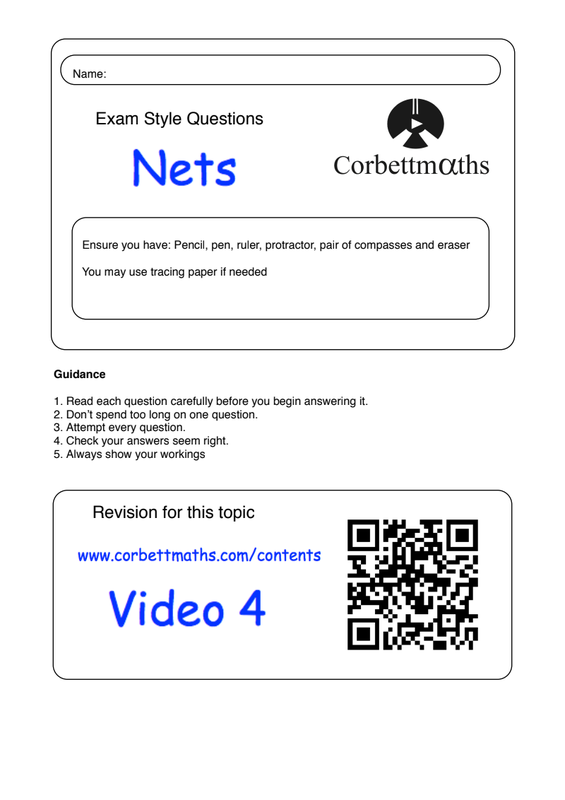2d shapes - 3d Nets of objects ANSWERS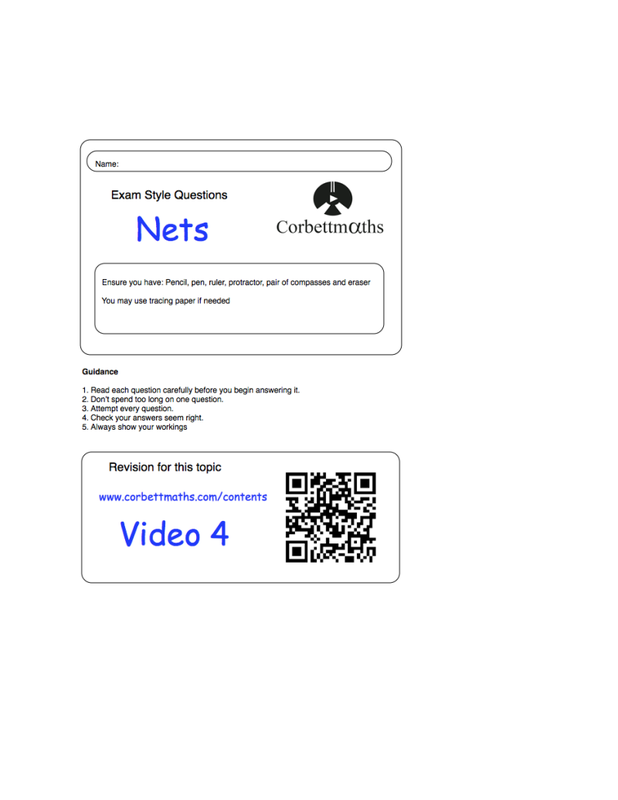2d 3d shapes - mixed questions sheet Hand Out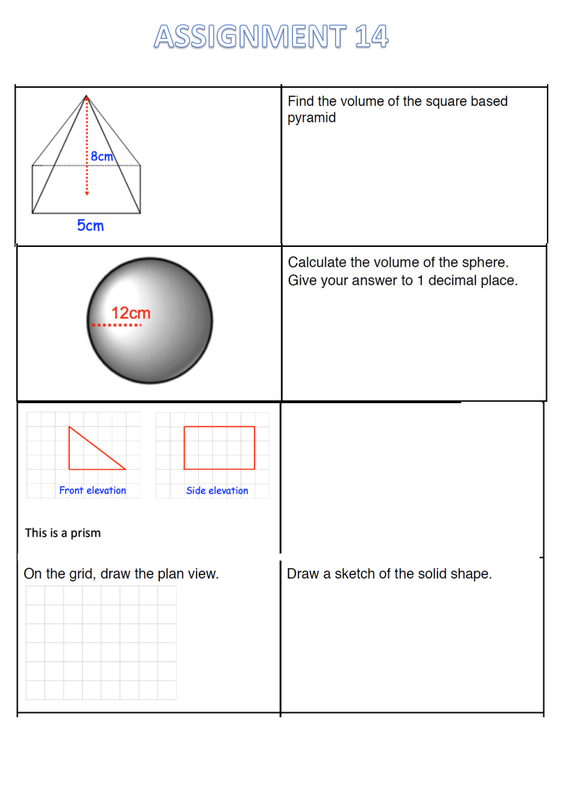3d shapes - Formula Hand out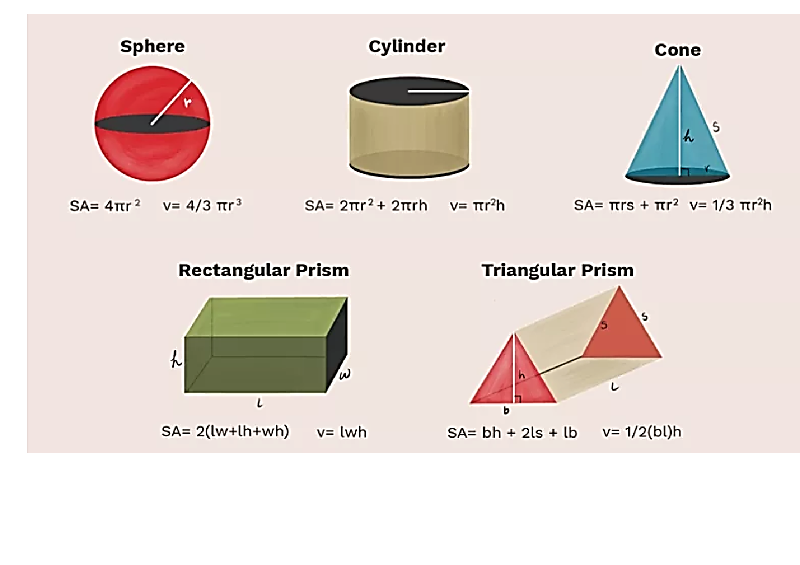3d shapes - Volume ppt 2021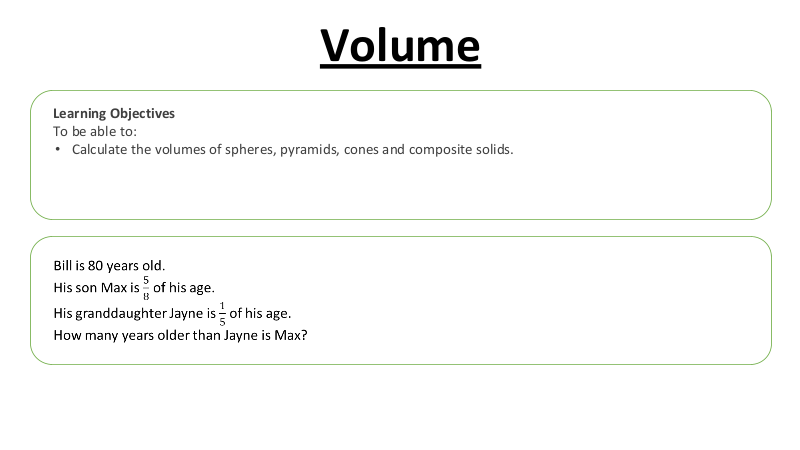3d shapes - Volume of a Cone Worksheet